# Monty Hall problem, with Thompson sampling

We all know the Monty Hall problem. Recently, Jason Rosenhouse published a book on that topic (entitled The Monty Hall Problem, The Remarkable Story of Math’s Most Contentious Brain Teaser). The game is more or less described by the following question

Suppose you’re on a game show, and you’re given the choice of three doors: Behind one door is a car; behind the others, goats. You pick a door, say No. 1, and the host, who knows what’s behind the doors, opens another door, say No. 3, which has a goat. He then says to you, “Do you want to pick door No. 2?” Is it to your advantage to switch your choice?

While I was preparing some slides for a lecture on Bayesian modeling and thinking, I wanted to find an illustration of what is sometimes called the Bayesian brain, that can be related to updates of beliefs, when we experience. And I was looking for examples of Thompson sampling. And actually, it is possible to learn that switching is the optimal strategy, in the Monty Hall problem, just by playing sequentially the game, and learning from previous strategies. The following code is used, to choose the door with the price (the car), and the one we first select

set.seed(1) n = 5000 listdoor = matrix(1:3,3,n) door = listdoor win = sample(1:3,size=n,replace=TRUE) pick1 = sample(1:3,size=n,replace=TRUE)

Then, the presenter picks one, that is neither the car, nor the one we chose initially. The following trick can be used, to get the list of available choices

door[win+(0:(n-1))*3] = NA door[,1:10] [,1] [,2] [,3] [,4] [,5] [,6] [,7] [,8] [,9] [,10] [1,] NA NA NA 1 NA NA 1 NA 1 NA [2,] 2 2 NA NA 2 2 2 NA NA 2 [3,] 3 NA 3 3 NA NA NA 3 NA NA door[pick1+(0:(n-1))*3] = NA door[,1:10] [,1] [,2] [,3] [,4] [,5] [,6] [,7] [,8] [,9] [,10] [1,] NA NA NA 1 NA NA 1 NA 1 NA [2,] 2 2 NA NA 2 2 2 NA NA 2 [3,] 3 NA 3 3 NA NA NA 3 NA NA

Then, the presenter picks one

presenter = apply(door,2, function(x) sample(x[!is.na(x)],size=1)) &gt; presenter[win != pick1] = apply(door,2,function(x) x[!is.na(x)])[win != pick1] presenter = unlist(presenter) presenter[1:10]  3 2 3 1 2 2 2 3 1 2

Now, let us consider the  Monty Hall problem. We have two possible strategies. The first one is to keep the door we chose, initially

pick2a = pick1 gaina = (pick2a==win) mean(gaina)  0.3392

As expected, on average, we win with (about) 1 chance out of 3. The second one is to (always) pick the other door (the one left). The code is close to the one we used before

door = listdoor door[pick1+(0:(n-1))*3] = NA door[presenter+(0:(n-1))*3] = NA pick2b = apply(door,2,function(x) x[!is.na(x)]) gainb = (pick2b==win) mean(gainb)  0.6608

If you know Monty Hall problem the probability to win is now 2 chance out of 3 (which is what the maths tells us). That is what we have with simulations.

Now, what if we don’t know how to do the maths, and we don’t want to compute it? We can use Thompson sampling to explore, and exploit. In a general context, we have to choose among On a le choix entre $K$ alternatives (here $K=2$, since we can either keep our initial choice, or pick the other one), and the output is $\boldsymbol{X}=(X_1,\cdots, X_K)$, where $X_k\sim\mathcal{B}(\theta_k)$, but $\theta_k$ is unknow, and we will play the game, and learn. From previous computations, we know that $\theta_1=1/3$ while $\theta_2=2/3$.

We use some prior distribution, $\theta_k\sim\mathcal{B}eta(\alpha_k,\beta_k)$, since the Beta distribution is the conjugate of the Bernoulli. At time $t$, we draw $K$ (independent) Beta variables $B_k\sim\mathcal{B}eta(\alpha_k,\beta_k)$, and pick $k^\star = \displaystyle{\underset{k=1,\cdots,K}{\text{argmax}}\{B_k\}}$.  Here the code will be

set.seed(2) X = cbind(pick2a == win,pick2b == win)*1 AB1 = AB2 = tirage = matrix(NA,n,2) choix = rep(NA,n) k=1 AB1[k,] = AB2[k,] = c(1,1) for(k in 1:(n-1)){ tirage[k,] = c(rbeta(1,AB1[k,1],AB1[k,2]), rbeta(1,AB2[k,1],AB2[k,2])) choix[k] = which.max(tirage[k,]) if(choix[k] == 1){ AB1[k+1,] = AB1[k,] + c(X[k,1],1-X[k,1]) AB2[k+1,] = AB2[k,] } if(choix[k] == 2){ AB1[k+1,] = AB1[k,] AB2[k+1,] = AB2[k,] + c(X[k,2],1-X[k,2]) }}

Before showing some graphs, let us check that indeed, we select more the second strategy (which is here to select the other door)

AB1[n,]  5 13 AB2[n,]  3292 1693

Indeed, since the average of a Beta distribution, $\mathcal{B}eta(\alpha,\beta)$ is $\alpha/(\alpha+\beta)$

AB2[n,1]/(sum(AB2[n,]))  0.6603811

i.e. the probability to win, with this second strategy is about $2/3$ (as obtained previously). We can visualize this on the animation below, with, in red the first strategy (keep your initial choice), in green the second one (select the other door), 0 and 1 respectively if we win, or not. Then we can visualize the evolution of $\alpha_2$ and $\beta_2$ on topc, and $\alpha_1$ and $\beta_1$ below (the index is time $t$). Finallly, we have the two variables $B_1$ and $B_2$ drawn,Of course, another simulation would have given different $B_1$‘s and $B_2$‘s, but finally, we learn that the second strategy is better, and we learn it quite fast…Here is another one (just to confirm)So clearly, even if we don’t know which is the optimal strategy (keep our initial choice, or switch), a player who played that game about 30 times should be able to understand that switching should be a better strategy.

# Classification from scratch, linear discrimination 8/8

Eighth post of our series on classification from scratch. The latest one was on the SVM, and today, I want to get back on very old stuff, with here also a linear separation of the space, using Fisher’s linear discriminent analysis.

## Bayes (naive) classifier

Consider the follwing naive classification rule$$m^\star(\mathbf{x})=\text{argmin}_y\{\mathbb{P}[Y=y\vert\mathbf{X}=\mathbf{x}]\}$$or$$m^\star(\mathbf{x})=\text{argmin}_y\left\{\frac{\mathbb{P}[\mathbf{X}=\mathbf{x}\vert Y=y]}{\mathbb{P}[\mathbf{X}=\mathbf{x}]}\right\}$$(where $\mathbb{P}[\mathbf{X}=\mathbf{x}]$ is the density in the continuous case).

In the case where $y$ takes two values, that will be standard $\{0,1\}$ here, one can rewrite the later as$$m^\star(\mathbf{x})=\begin{cases}1\text{ if }\mathbb{E}(Y\vert \mathbf{X}=\mathbf{x})>\displaystyle{\frac{1}{2}}\\0\text{ otherwise}\end{cases}$$and the set$$\mathcal{D}_S =\left\{\mathbf{x},\mathbb{E}(Y\vert \mathbf{X}=\mathbf{x})=\frac{1}{2}\right\}$$is called the decision boundary.

Assume that$$\mathbf{X}\vert Y=0\sim\mathcal{N}(\mathbf{\mu}_0,\mathbf{\Sigma})$$and$$\mathbf{X}\vert Y=1\sim\mathcal{N}(\mathbf{\mu}_1,\mathbf{\Sigma})$$then explicit expressions can be derived.$$m^\star(\mathbf{x})=\begin{cases}1\text{ if }r_1^2< r_0^2+2\displaystyle{\log\frac{\mathbb{P}(Y=1)}{\mathbb{P}(Y=0)}+\log\frac{\vert\mathbf{\Sigma}_0\vert}{\vert\mathbf{\Sigma}_1\vert}}\\0\text{ otherwise}\end{cases}$$where $r_y^2$ is the Manalahobis distance, $$r_y^2 = [\mathbf{X}-\mathbf{\mu}_y]^{\text{{T}}}\mathbf{\Sigma}_y^{-1}[\mathbf{X}-\mathbf{\mu}_y]$$

Let $\delta_y$be defined as$$\delta_y(\mathbf{x})=-\frac{1}{2}\log\vert\mathbf{\Sigma}_y\vert-\frac{1}{2}[{\color{blue}{\mathbf{x}}}-\mathbf{\mu}_y]^{\text{{T}}}\mathbf{\Sigma}_y^{-1}[{\color{blue}{\mathbf{x}}}-\mathbf{\mu}_y]+\log\mathbb{P}(Y=y)$$the decision boundary of this classifier is $$\{\mathbf{x}\text{ such that }\delta_0(\mathbf{x})=\delta_1(\mathbf{x})\}$$which is quadratic in ${\color{blue}{\mathbf{x}}}$. This is the quadratic discriminant analysis. This can be visualized bellow.The decision boundary is hereBut that can’t be the linear discriminant analysis, right? I mean, the frontier is not linear… Actually, in Fisher’s seminal paper, it was assumed that $\mathbf{\Sigma}_0=\mathbf{\Sigma}_1$.

In that case, actually, $$\delta_y(\mathbf{x})={\color{blue}{\mathbf{x}}}^{\text{T}}\mathbf{\Sigma}^{-1}\mathbf{\mu}_y-\frac{1}{2}\mathbf{\mu}_y^{\text{T}}\mathbf{\Sigma}^{-1}\mathbf{\mu}_y+\log\mathbb{P}(Y=y)$$ and the decision frontier is now linear in ${\color{blue}{\mathbf{x}}}$. This is the linear discriminant analysis. This can be visualized bellowHere the two samples have the same variance matrix and the frontier is## Link with the logistic regression

Assume as previously that$$\mathbf{X}\vert Y=0\sim\mathcal{N}(\mathbf{\mu}_0,\mathbf{\Sigma})$$and$$\mathbf{X}\vert Y=1\sim\mathcal{N}(\mathbf{\mu}_1,\mathbf{\Sigma})$$then$$\log\frac{\mathbb{P}(Y=1\vert \mathbf{X}=\mathbf{x})}{\mathbb{P}(Y=0\vert \mathbf{X}=\mathbf{x})}$$is equal to $$\mathbf{x}^{\text{{T}}}\mathbf{\Sigma}^{-1}[\mathbf{\mu}_y]-\frac{1}{2}[\mathbf{\mu}_1-\mathbf{\mu}_0]^{\text{{T}}}\mathbf{\Sigma}^{-1}[\mathbf{\mu}_1-\mathbf{\mu}_0]+\log\frac{\mathbb{P}(Y=1)}{\mathbb{P}(Y=0)}$$which is linear in $\mathbf{x}$$$\log\frac{\mathbb{P}(Y=1\vert \mathbf{X}=\mathbf{x})}{\mathbb{P}(Y=0\vert \mathbf{X}=\mathbf{x})}=\mathbf{x}^{\text{{T}}}\mathbf{\beta}$$Hence, when each groups have Gaussian distributions with identical variance matrix, then LDA and the logistic regression lead to the same classification rule.

Observe furthermore that the slope is proportional to $\mathbf{\Sigma}^{-1}[\mathbf{\mu}_1-\mathbf{\mu}_0]$, as stated in Fisher’s article. But to obtain such a relationship, he observe that the ratio of between and within variances (in the two groups) was$$\frac{\text{variance between}}{\text{variance within}}=\frac{[\mathbf{\omega}\mathbf{\mu}_1-\mathbf{\omega}\mathbf{\mu}_0]^2}{\mathbf{\omega}^{\text{T}}\mathbf{\Sigma}_1\mathbf{\omega}+\mathbf{\omega}^{\text{T}}\mathbf{\Sigma}_0\mathbf{\omega}}$$which is maximal when $\mathbf{\omega}$ is proportional to $\mathbf{\Sigma}^{-1}[\mathbf{\mu}_1-\mathbf{\mu}_0]$, when $\mathbf{\Sigma}_0=\mathbf{\Sigma}_1$.

## Homebrew linear discriminant analysis

To compute vector $\mathbf{\omega}$

m0 = apply(myocarde[myocarde$PRONO=="0",1:7],2,mean) m1 = apply(myocarde[myocarde$PRONO=="1",1:7],2,mean) Sigma = var(myocarde[,1:7]) omega = solve(Sigma)%*%(m1-m0) omega [,1] FRCAR -0.012909708542 INCAR 1.088582058796 INSYS -0.019390084344 PRDIA -0.025817110020 PAPUL 0.020441287970 PVENT -0.038298291091 REPUL -0.001371677757

For the constant – in the equation $\omega^T\mathbf{x}+b=0$ – if we have equiprobable probabilities, use

b = (t(m1)%*%solve(Sigma)%*%m1-t(m0)%*%solve(Sigma)%*%m0)/2

## Application (on the small dataset)

In order to visualize what’s going on, consider the small dataset, with only two covariates,

x = c(.4,.55,.65,.9,.1,.35,.5,.15,.2,.85) y = c(.85,.95,.8,.87,.5,.55,.5,.2,.1,.3) z = c(1,1,1,1,1,0,0,1,0,0) df = data.frame(x1=x,x2=y,y=as.factor(z)) m0 = apply(df[df$y=="0",1:2],2,mean) m1 = apply(df[df$y=="1",1:2],2,mean) Sigma = var(df[,1:2]) omega = solve(Sigma)%*%(m1-m0) omega [,1] x1 -2.640613174 x2 4.858705676Using R regular function, we get

library(MASS) fit_lda = lda(y ~x1+x2 , data=df) fit_lda   Coefficients of linear discriminants: LD1 x1 -2.588389554 x2 4.762614663

which is the same coefficient as the one we got with our own code. For the constant, use

b = (t(m1)%*%solve(Sigma)%*%m1-t(m0)%*%solve(Sigma)%*%m0)/2

If we plot it, we get the red straight line

plot(df$x1,df$x2,pch=c(1,19)[1+(df$y=="1")]) abline(a=b/omega,b=-omega/omega,col="red")As we can see (with the blue points), our red line intersects the middle of the segment of the two barycenters points(m0["x1"],m0["x2"],pch=4) points(m1["x1"],m1["x2"],pch=4) segments(m0["x1"],m0["x2"],m1["x1"],m1["x2"],col="blue") points(.5*m0["x1"]+.5*m1["x1"],.5*m0["x2"]+.5*m1["x2"],col="blue",pch=19) Of course, we can also use R function predlda = function(x,y) predict(fit_lda, data.frame(x1=x,x2=y))$class==1 vv=outer(vu,vu,predlda) contour(vu,vu,vv,add=TRUE,lwd=2,levels = .5)One can also consider the quadratic discriminent analysis since it might be difficult to argue that $\mathbf{\Sigma}_0=\mathbf{\Sigma}_1$

fit_qda = qda(y ~x1+x2 , data=df)

The separation curve is here

plot(df$x1,df$x2,pch=19, col=c("blue","red")[1+(df$y=="1")]) predqda=function(x,y) predict(fit_qda, data.frame(x1=x,x2=y))$class==1 vv=outer(vu,vu,predlda) contour(vu,vu,vv,add=TRUE,lwd=2,levels = .5)# How long could it take to run a regression

This afternoon, while I was discussing with Montserrat (aka @mguillen_estany) we were wondering how long it might take to run a regression model. More specifically, how long it might take if we use a Bayesian approach. My guess was that the time should probably be linear in , the number of observations. But I thought I would be good to check.

Let us generate a big dataset, with one million rows,

> n=1e6
> X=runif(n)
> Y=2+5*X+rnorm(n)
> B=data.frame(X,Y)

Consider as a benchmark the standard linear regression,

> lm_freq = function(n){
+   idx = sample(1:1e6,size=n)
+   reg = lm(Y~X,data=B[idx,])
+   summary(reg)
+ }

Here the regression is a subset of smaller size. We can do the same with a Bayesian approach, using stan,

> stan_lm ="
+ data {
+ int N;
+ vector[N] x;
+ vector[N] y;
+ }
+ parameters {
+ real alpha;
+ real beta;
+ real tau;
+ }
+ transformed parameters {
+ real sigma;
+ sigma <- 1 / sqrt(tau);
+ }
+ model{
+ y ~ normal(alpha + beta * x, sigma);
+ alpha ~ normal(0, 10);
+ beta ~ normal(0, 10);
+ tau ~ gamma(0.001, 0.001);
+ }
+ "

Define then the model

> library(rstan)
> system.time(
stanmodel <<- stan_model(model_code = stan_lm))
utilisateur     système      écoulé
0.043       0.000       0.043

We want to see how long it might take to run a regression,

> lm_bayes = function(n){
+   idx = sample(1:1e6,size=n)
+   fit = sampling(stanmodel,
+       data = list(N=n,
+                   x=X[idx],
+                   y=Y[idx]),
+       iter = 1000, warmup=200)
+   summary(fit)
+ }

We use the following package to see how long it takes

> library(microbenchmark)
> time_lm = function(n){
+  M = microbenchmark(lm_freq(n),
+      lm_bayes(n),times=50)
+  return(apply( matrix(M$time,nrow=2),1,mean)) + } We can now compare the time it took with ten, one hundred, on thousand, and ten thousand observations, > vN = c(10,100,1000,10000) > T = Vectorize(time_lm)(vN) we can then plot it > plot(vN,T[2,]/1e6,log="xy",col="red",type="b", + xlab="Number of Observations",ylab="Time") > lines(vN,T[1,]/1e6,col="blue",type="b")It looks like (if we forget about the very small sample) that the time it takes to run a regression is linear, with the two techniques (the frequentist and the Bayesian ones). And actually, the same story olds for logistic regressions. Consider the following dataset > n=1e6 > X=runif(n) > S=-3+2*X+rnorm(n) > Y=rbinom(n,size=1,prob=exp(S)/(1+exp(S))) > B=data.frame(X,Y) The frequentist version of the logistic regression is > glm_freq = function(n){ + idx = sample(1:1e6,size=n) + reg = glm(Y~X,data=B[idx,],family=binomial) + summary(reg) + } and the Bayesian one, using stan, > stan_glm = " + data { + int N; + vector[N] x; + int<lower=0,upper=1> y[N]; + } + parameters { + real alpha; + real beta; + } + model { + alpha ~ normal(0, 10); + beta ~ normal(0, 10); + y ~ bernoulli_logit(alpha + beta * x); + } + " > stanmodel = stan_model(model_code = stan_glm) ) > glm_bayes = function(n){ + idx = sample(1:1e6,size=n) + fit = sampling(stanmodel, + data = list(N=n, + x = X[idx], + y = Y[idx]), + iter = 1000, warmup=200) + summary(fit) + } Again, we can see how long it takes to run those regression models > time_gl m= function(n){ + M = microbenchmark(glm_freq(n), + glm_bayes(n),times=50) + return(apply( matrix(M$time,nrow=2),1,mean))
+ }# Will I ever be a bayesian statistician ? (part 1)

Last week, during the workshop on Statistical Methods for Meteorology and Climate Change (here), I discovered how powerful bayesian techniques could be, and that there were more and more bayesian statisticians. So, if I was to fully understand applied statisticians in conferences and workshops, I really have to understand basics of bayesian statistics. I have published some time ago some posts on bayesian statistics applied to actuarial problems (here or there), but so far, I always thought that bayesian was a synonym for magician. To be honest, I am a Muggle, and I have not been trained as a bayesian. But I can be an opportunist…

So I decided to publish some posts on bayesian techniques, in order to prove that it is actually not that difficult to implement.As far as I understand it, in bayesian statistics, the parameter is considered as a random variable (which is also the case, in classical mathematical statistics). But here, here assume that this parameter does have a parametric distribution….
Consider a classical statistical problem: assume we have a sample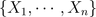i.i.d. with distribution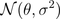. Here we note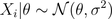since parameteris a random variable. The idea is to assume thathas a (so called a priori) distribution, e.g.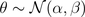So far it was simple. The idea is then to consider the posterior distribution of, given the observations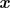. Thus, we need to compute the distribution of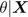which is here extremely simple (due to properties of the Gaussian distribution), i.e.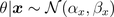where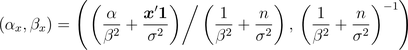And them, it becomes extremely natural to consider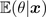as an estimator of given our sample data (and thus, we also have a confidence interval since we know the distribution ofgiven the observations).
In order to be sure that we understood, consider now a heads and tails problem, i.e.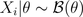. Note, first, that \theta has support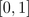. So we need a distribution on that support. Why not a beta distribution ? E.g.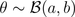Thus,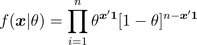and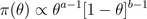From Bayes formula,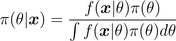and we get easily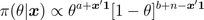which is the density of a Beta distribution, i.e.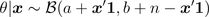prior=dbeta(u,a,b)
posterior=dbeta(u,a+y,n-y+b)

The estimator proposed is then the expected value of that conditional distribution,Note that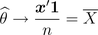Further, it is possible to derive confidence intervals using quantiles of the posterior distribution.
On the graphs below, we consider the following heads/tails sampleA first idea is to consider a uniform prior distribution.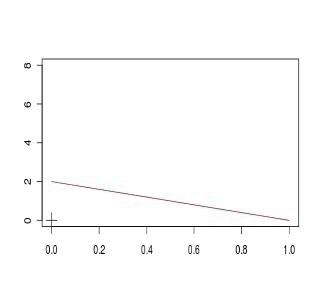A second idea is to consider an asymmetric beta distribution. First, with an asymmetry on the left,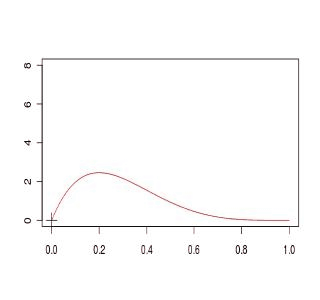or on the right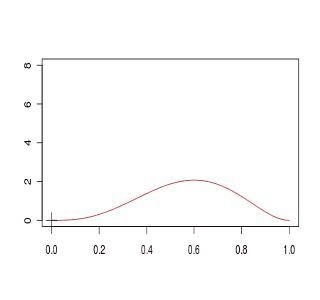Finally a third idea is simply to get back to the standard Gaussian approximation,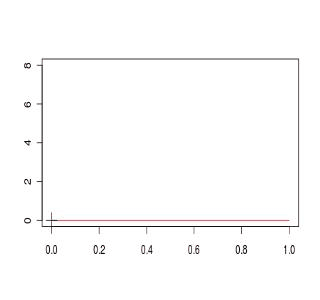If we compare the four models, we obtain (the plain black line is the Gaussian approximated distribution for the empirical mean), and red lines are obtained from prior beta distributionsThe code to generate those graphs is the following
a1=1; b1=1
D1[1,]=dbeta(u,a,b)
a2=4; b2=2
D2[1,]=dbeta(u,a,b)
a3=2; b3=4
D3[1,]=dbeta(u,a,b)
setseed(1)
S=sample(0:1,size=100,replace=TRUE)
COULEUR=rev(rainbow(120))
D1=D2=D3=D4=matrix(NA,101,length(u))
for(s in 1:100){
y=sum(S[1:s])
D1[s+1,]=dbeta(u,a1+y,s-y+b1)
D2[s+1,]=dbeta(u,a2+y,s-y+b2)
D3[s+1,]=dbeta(u,a3+y,s-y+b3)
D4[s+1,]=dnorm(u,y/s,sqrt(y/s*(1-y/s)/s))
plot(u,D1[1,],col="black",type="l",ylim=c(0,8),
xlab="",ylab="")
for(i in 1:s){lines(u,D1[1+i,],col=COULEUR[i])}
points(y/s,0,pch=3,cex=2)
plot(u,D2[1,],col="black",type="l",ylim=c(0,8),
xlab="",ylab="")
for(i in 1:s){lines(u,D2[1+i,],col=COULEUR[i])}
points(y/s,0,pch=3,cex=2)
plot(u,D3[1,],col="black",type="l",ylim=c(0,8),
xlab="",ylab="")
for(i in 1:s){lines(u,D3[1+i,],col=COULEUR[i])}
points(y/s,0,pch=3,cex=2)
plot(u,D4[1,],col="white",type="l",ylim=c(0,8),
xlab="",ylab="")
for(i in 1:s){lines(u,D4[1+i,],col=COULEUR[i])}
points(y/s,0,pch=3,cex=2)
plot(u,D4[s+1,],col="black",lwd=2,type="l",
ylim=c(0,8),xlab="",ylab="")
lines(u,D1[1+i,],col="blue")
lines(u,D2[1+i,],col="red")
lines(u,D3[1+i,],col="purple")
points(y/s,0,pch=3,cex=2)
}

Here, we can see that computations are simple if the prior distribution has a distribution which is the conjugate of the observations’ distribution (see here for the list of prior and posterior standard distributions).
So far, I have two questions that naturally show up

• is it possible to start with a neutral prior distribution, non informative ?
• what if we are no longer working with conjugate distributions ?

Well, I guess I have to work a bit more to answer those questions…. to be continued